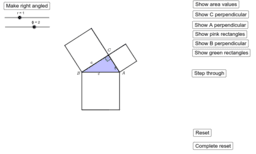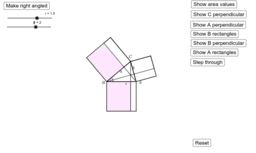# Generalising Pythagoras

Author:
Jim Simons
This is about a generalisation of Pythagoras's theorem that applies to a general triangle. It is is roughly equivalent to the cosine rule, but is purely geometric, about the areas if rectangles. There is no trigonometry in its statement or proof.
•### Demonstrate generalisation of Pythagoras

•### First proof of Pythagoras generalisation

•